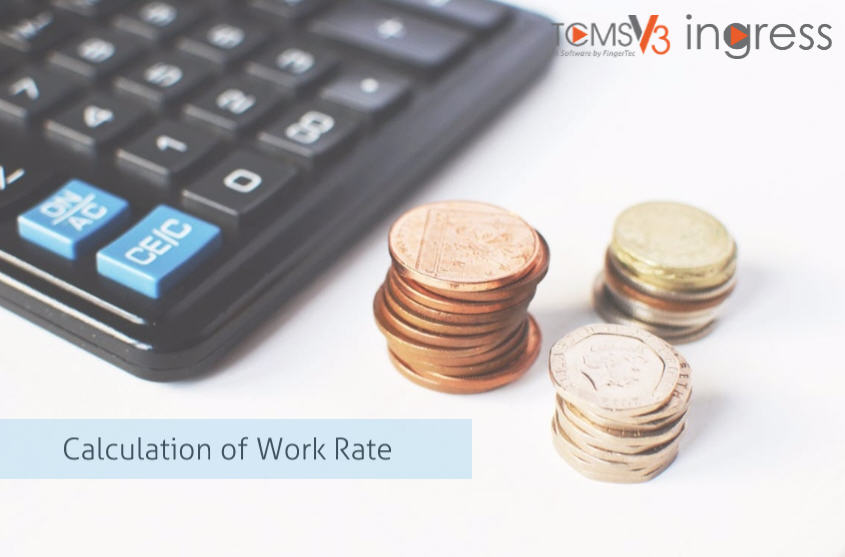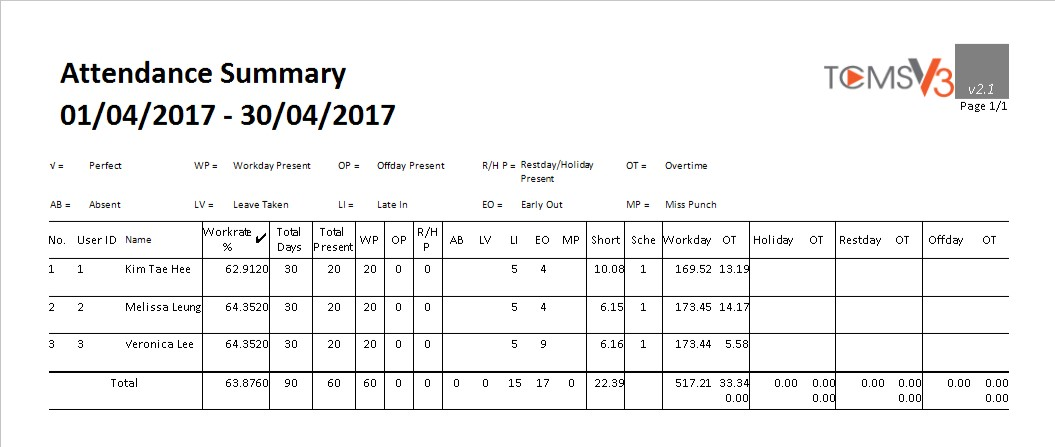# Calculation Formula in Ingress & TCMS V3 Reports Friday, June 16, 2017 FingerTec 0 CommentsIntroduction
Rates of the employee attendance including the rate of absenteeism are the indications of the workplace environment and satisfaction of employees. By calculating these rates, the employer can determine how well your employees’ performance in the company because a low rate attendance will decrease the productivity of the company.

Benefit
From this calculation, it will display the average attendance performance of each employee.Calculation of Work Rate
Work Rate = [(total of actual worked hours) / (total offer hours)] x 100%
Total days   = 30
Total hours = 30 days x 9 hours per day
= 270 hours.
Total of actual worked hours = 169.52 as written in WORKDAY column
= 169 hour 52 min
= 169.87 hours
Work Rate = [169.87 / 270 ] x 100%
= 62.91% (same as printed in report)

NOTE:
Work rate calculation is applied in Attendance Summary, Day-by-Day Analysis and Month-by-Month Analysis.